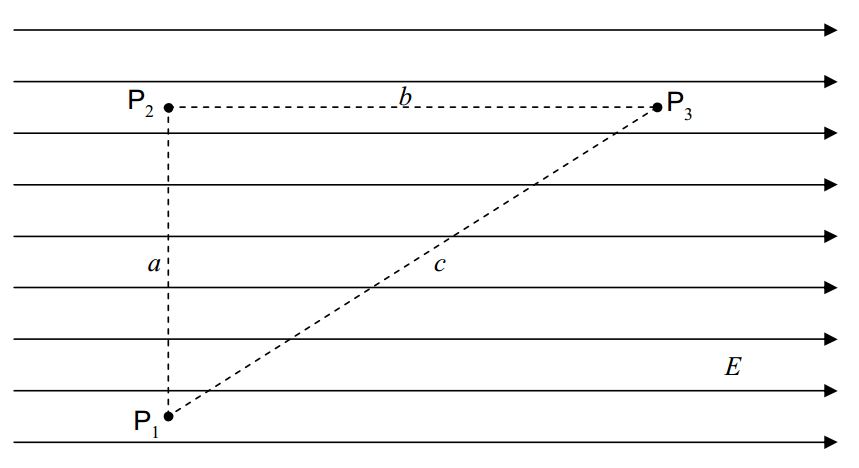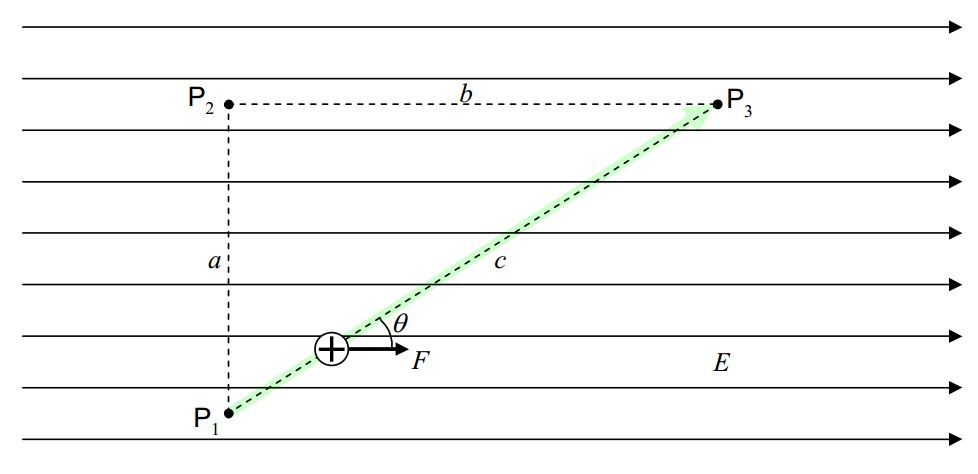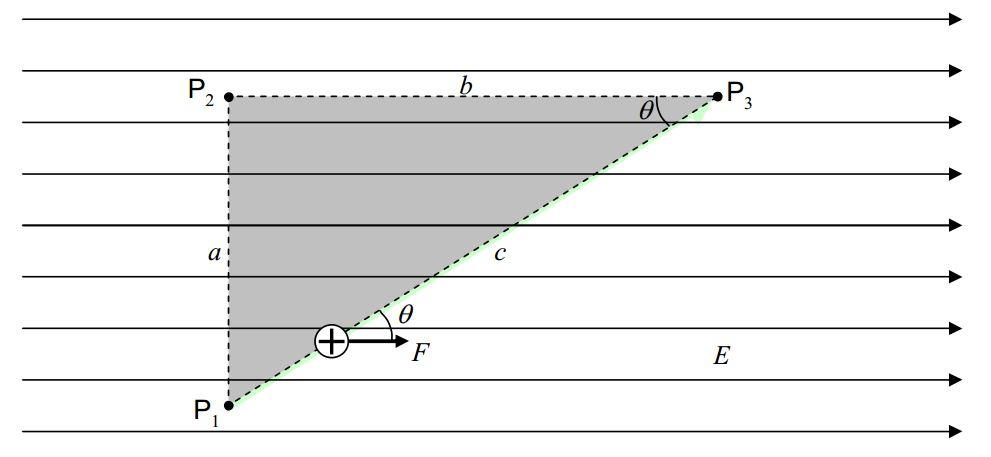# B5: Work Done by the Electric Field and the Electric Potential

$$\newcommand{\vecs}{\overset { \rightharpoonup} {\mathbf{#1}} }$$ $$\newcommand{\vecd}{\overset{-\!-\!\rightharpoonup}{\vphantom{a}\smash {#1}}}$$$$\newcommand{\id}{\mathrm{id}}$$ $$\newcommand{\Span}{\mathrm{span}}$$ $$\newcommand{\kernel}{\mathrm{null}\,}$$ $$\newcommand{\range}{\mathrm{range}\,}$$ $$\newcommand{\RealPart}{\mathrm{Re}}$$ $$\newcommand{\ImaginaryPart}{\mathrm{Im}}$$ $$\newcommand{\Argument}{\mathrm{Arg}}$$ $$\newcommand{\norm}{\| #1 \|}$$ $$\newcommand{\inner}{\langle #1, #2 \rangle}$$ $$\newcommand{\Span}{\mathrm{span}}$$ $$\newcommand{\id}{\mathrm{id}}$$ $$\newcommand{\Span}{\mathrm{span}}$$ $$\newcommand{\kernel}{\mathrm{null}\,}$$ $$\newcommand{\range}{\mathrm{range}\,}$$ $$\newcommand{\RealPart}{\mathrm{Re}}$$ $$\newcommand{\ImaginaryPart}{\mathrm{Im}}$$ $$\newcommand{\Argument}{\mathrm{Arg}}$$ $$\newcommand{\norm}{\| #1 \|}$$ $$\newcommand{\inner}{\langle #1, #2 \rangle}$$ $$\newcommand{\Span}{\mathrm{span}}$$$$\newcommand{\AA}{\unicode[.8,0]{x212B}}$$

When a charged particle moves from one position in an electric field to another position in that same electric field, the electric field does work on the particle. The work done is conservative; hence, we can define a potential energy for the case of the force exerted by an electric field. This allows us to use the concepts of work, energy, and the conservation of energy, in the analysis of physical processes involving charged particles and electric fields.

We have defined the work done on a particle by a force, to be the force-along-the-path times the length of the path, with the stipulation that when the component of the force along the path is different on different segments of the path, one has to divide up the path into segments on each of which the force-along-the-path has one value for the whole segment, calculate the work done on each segment, and add up the results.

Let’s investigate the work done by the electric field on a charged particle as it moves in the electric field in the rather simple case of a uniform electric field. For instance, let’s calculate the work done on a positively-charged particle of charge q as it moves from point $$P_1$$ to point $$P_3$$along the path: “From $$P_1$$ straight to point $$P_2$$ and from there, straight to $$P_3$$.” Note that we are not told what it is that makes the particle move. We don’t care about that in this problem. Perhaps the charged particle is on the end of a quartz rod (quartz is a good insulator) and a person who is holding the rod by the other end moves the rod so the charged particle moves as specified.

Along the first part of the path, from $$P_1$$ to $$P_2$$, the force on the charged particle is perpendicular to the path.The force has no component along the path so it does no work on the charged particle at all as the charged particle moves from point $$P_1$$ to point $$P_2$$.

$W_{12}=0 \nonumber$

From $$P_2$$, the particle goes straight to $$P_3$$.

On that segment of the path (from $$P_2$$ to $$P_3$$ ) the force is in exactly the same direction as the direction in which the particle is going.As such, the work is just the magnitude of the force times the length of the path segment:

$W_{23}=Fb \nonumber$

The magnitude of the force is the charge of the particle times the magnitude of the electric field $$F = qE$$, so,

$W_{23}=qEb \nonumber$

Thus, the work done on the charged particle by the electric field, as the particle moves from point $$P_1$$ to $$P_3$$ along the specified path is

$W_{123}=W_{12}+W_{23} \nonumber$

$W_{123}=0+qEb \nonumber$

$W_{123}=qEb \nonumber$

Now let’s calculate the work done on the charged particle if it undergoes the same displacement (from $$P_1$$ to $$P_3$$ ) but does so by moving along the direct path, straight from $$P_1$$ to $$P_3$$.The force on a positively-charged particle being in the same direction as the electric field, the force vector makes an angle $$\theta$$ with the path direction and the expression

$W=\vec{F} \cdot \vec{\Delta r} \nonumber$

for the work becomes

$W_{13}=F c \, cos \theta \nonumber$

$W_{13}=qE c \, cos \theta \nonumber$

Analyzing the shaded triangle in the following diagram:we find that $$cos \theta=\frac{b}{c}$$. Substituting this into our expression for the work ( $$W_{13}=qE c \, cos \theta$$ ) yields

$W_{13}=qEc \frac{b}{c} \nonumber$

$W_{13}=qEb \nonumber$

This is the same result we got for the work done on the charged particle by the electric field as the particle moved between the same two points (from $$P_1$$ to $$P_3$$ ) along the other path ($$P_1$$ to $$P_2$$ to $$P_3$$ ). As it turns out, the work done is the same no matter what path the particle takes on its way from $$P_1$$ to $$P_3$$. I don’t want to take the time to prove that here but I would like to investigate one more path (not so much to get the result, but rather, to review an important point about how to calculate work). Referring to the diagram:Let’s calculate the work done on a particle with charge $$q$$, by the electric field, as the particle moves from $$P_1$$ to $$P_3$$ along the path “from $$P_1$$ straight to $$P_4$$, from $$P_4$$ straight to $$P_5$$, and from $$P_5$$ straight to $$P_3$$.” On $$P_1$$ to $$P_4$$, the force is in the exact same direction as the direction in which the particle moves along the path, so,

$W_{14}=F(b+d) \nonumber$

$W_{14}=qE(b+d) \nonumber$

From point $$P_4$$ to $$P_5$$, the force exerted on the charged particle by the electric field is at right angles to the path, so, the force does no work on the charged particle on segment $$P_4$$ to $$P_5$$.

$W_{45}=0 \nonumber$

On the segment from $$P_5$$ to $$P_3$$,the force is in the exact opposite direction to the direction in which the particle moves. This means that the work done by the force of the electric field on the charged particle as the particle moves form $$P_5$$ to $$P_3$$ is the negative of the magnitude of the force times the length of the path segment. Thus

$W_{53}=-Fd \nonumber$

$W_{53}=-qEd \nonumber$

and

$W_{1453}=W_{14}+W_{45}+W_{53} \nonumber$

$W_{1453}=qE(b+d)+0+(-qEd) \nonumber$

$W_{1453}=qEb \nonumber$

As advertised, we obtain the same result for the work done on the particle as it moves from $$P_1$$ to $$P_3$$ along “$$P_1$$ to $$P_4$$ to $$P_5$$ to $$P_3$$” as we did on the other two paths.

Whenever the work done on a particle by a force acting on that particle, when that particle moves from point $$P_1$$ to point $$P_3$$, is the same no matter what path the particle takes on the way from $$P_1$$ to $$P_3$$, we can define a potential energy function for the force. The potential energy function is an assignment of a value of potential energy to every point in space. Such an assignment allows us to calculate the work done on the particle by the force when the particle moves from point $$P_1$$ to point $$P_3$$ simply by subtracting the value of the potential energy of the particle at $$P_1$$ from the value of the potential energy of the particle at $$P_3$$ and taking the negative of the result. In other words, the work done on the particle by the force of the electric field when the particle goes from one point to another is just the negative of the change in the potential energy of the particle.

In determining the potential energy function for the case of a particle of charge $$q$$ in a uniform electric field $$\vec{E}$$, (an infinite set of vectors, each pointing in one and the same direction and each having one and the same magnitude $$E$$ ) we rely heavily on your understanding of the nearearth’s-surface gravitational potential energy. Near the surface of the earth, we said back in volume 1 of this book, there is a uniform gravitational field, (a force-per-mass vector field) in the downward direction. A particle of mass $$m$$ in that field has a force “$$mg$$ downward” exerted upon it at any location in the vicinity of the surface of the earth. For that case, the potential energy of a particle of mass $$m$$ is given by $$mgy$$ where $$mg$$ is the magnitude of the downward force and $$y$$ is the height that the particle is above an arbitrarily-chosen reference level. For ease of comparison with the case of the electric field, we now describe the reference level for gravitational potential energy as a plane, perpendicular to the gravitational field $$g$$, the force-per mass vector field; and; we call the variable $$y$$ the “upfield” distance (the distance in the direction opposite that of the gravitational field) that the particle is from the reference plane. (So, we’re calling the direction in which the gravitational field points, the direction you know to be downward, the “downfield” direction.)

Now let’s switch over to the case of the uniform electric field. As in the case of the near-earth’s surface gravitational field, the force exerted on its victim by a uniform electric field has one and the same magnitude and direction at any point in space. Of course, in the electric field case, the force is $$qE$$ rather than $$mg$$ and the characteristic of the victim that matters is the charge $$q$$ rather than the mass $$m$$. We call the direction in which the electric field points, the “downfield” direction, and the opposite direction, the “upfield” direction. Now we arbitrarily define a plane that is perpendicular to the electric field to be the reference plane for the electric potential energy of a particle of charge $$q$$ in the electric field. If we call $$d$$ the distance that the charged particle is away from the plane in the upfield direction, then the potential energy of the particle with charge $$q$$ is given by

$U=qEd \nonumber$

where

$$U$$ is the electric potential energy of the charged particle,

$$q$$ is the charge of the particle,

$$E$$ is the magnitude of every electric field vector making up the uniform electric field, and

$$d$$ is the “upfield” distance that the particle is from the $$U = 0$$ reference plane.

Let’s make sure this expression for the potential energy function gives the result we obtained previously for the work done on a particle with charge $$q$$, by the uniform electric field depicted in the following diagram, when the particle moves from $$P_1$$ to $$P_3$$As you can see, I have chosen (for my own convenience) to define the reference plane to be at the most downfield position relevant to the problem. With that choice, the particle of charge $$q$$, when it is at $$P_1$$ has potential energy $$qEb$$ (since point $$P_1$$ is a distance $$b$$ “upfield” from the reference plane) and, when it is at $$P_3$$, the particle of charge $$q$$ has potential energy $$0$$ since $$P_3$$ is on the reference plane.

$W_{13}=-\Delta U \nonumber$

$W_{13}=-(U_3-U_1) \nonumber$

$W_{13}=-(0-qEb) \nonumber$

$W_{13}=qEb \nonumber$

This is indeed the result we got (for the work done by the electric field on the particle with charge $$q$$ as that particle was moved from $$P_1$$ to $$P_3$$) the other three ways that we calculated this work.

This page titled B5: Work Done by the Electric Field and the Electric Potential is shared under a CC BY-SA 2.5 license and was authored, remixed, and/or curated by Jeffrey W. Schnick via source content that was edited to the style and standards of the LibreTexts platform; a detailed edit history is available upon request.